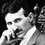# Help

What is the maximum value of $x^2 y^3$ for positive numbers $x$ and $y$ satisfying $x+y=2$?Note by Abhishek Alva
4 years, 8 months ago

This discussion board is a place to discuss our Daily Challenges and the math and science related to those challenges. Explanations are more than just a solution — they should explain the steps and thinking strategies that you used to obtain the solution. Comments should further the discussion of math and science.

When posting on Brilliant:

• Use the emojis to react to an explanation, whether you're congratulating a job well done , or just really confused .
• Ask specific questions about the challenge or the steps in somebody's explanation. Well-posed questions can add a lot to the discussion, but posting "I don't understand!" doesn't help anyone.
• Try to contribute something new to the discussion, whether it is an extension, generalization or other idea related to the challenge.
• Stay on topic — we're all here to learn more about math and science, not to hear about your favorite get-rich-quick scheme or current world events.

MarkdownAppears as
*italics* or _italics_ italics
**bold** or __bold__ bold
- bulleted- list
• bulleted
• list
1. numbered2. list
1. numbered
2. list
Note: you must add a full line of space before and after lists for them to show up correctly
paragraph 1paragraph 2

paragraph 1

paragraph 2

[example link](https://brilliant.org)example link
> This is a quote
This is a quote
    # I indented these lines
# 4 spaces, and now they show
# up as a code block.

print "hello world"
# I indented these lines
# 4 spaces, and now they show
# up as a code block.

print "hello world"
MathAppears as
Remember to wrap math in $$ ... $$ or $ ... $ to ensure proper formatting.
2 \times 3 $2 \times 3$
2^{34} $2^{34}$
a_{i-1} $a_{i-1}$
\frac{2}{3} $\frac{2}{3}$
\sqrt{2} $\sqrt{2}$
\sum_{i=1}^3 $\sum_{i=1}^3$
\sin \theta $\sin \theta$
\boxed{123} $\boxed{123}$

## Comments

Sort by:

Top Newest

Is it 3456/3125. ?

- 4 years, 8 months ago

Log in to reply

please write the solution

- 4 years, 8 months ago

Log in to reply

Am i correct?

- 4 years, 8 months ago

Log in to reply

yes u are write the solution

- 4 years, 8 months ago

Log in to reply

Apply Am gm on x,x,2y/3,2y/3,2y/3.

- 4 years, 8 months ago

Log in to reply

a/(a^3+b^2+c )+b/(b^3+c^2+a)+c/(c^3+a^2+b) if a+b+c=3 determine the largest value of the equation given that a b c are positive real numbers

- 4 years, 8 months ago

Log in to reply

i not getting this problem

- 4 years, 8 months ago

Log in to reply

I got the answer to be $\dfrac{676\sqrt{26}}{3125}$ which is very close to your answer.First we get the max. value of $xy$ to be $1$ and $x^2+y^2$ to be $2$ by $AM-GM$ inequality.Now we must find the max value of $2x+3y.$We have an inequality which states that $ax+by\leq \sqrt{a^2+b^2}\times\sqrt{x^2+y^2}.$Well I dont know the proof for this inequality.Hence, $2x+3y\leq\sqrt{2^2+3^2}\times\sqrt 2=\sqrt{26}.$ Next we apply AM-GM inequality for $2x+3y.$We split the terms and apply the inequality$.\dfrac{x+x+y+y+y}{5}\geq\sqrt{x^2y^3}\Rightarrow \dfrac{2x+3y}{5}\geq\sqrt{x^2y^3}.$Therefore,$\dfrac{\sqrt{26}}{5}\geq\sqrt{x^2y^3}.$Now raise both sides by power of $5.$We get the maximum value of $x^2y^3$ to be $\boxed{\dfrac{676\sqrt{26}}{3125}}$$.$

- 4 years, 8 months ago

Log in to reply

When you applied am gm on x,x,y,y,y the equality holds when x=y which implies that max value of x²y³ is 1.I think this was ur mistake @Ayush Rai.

- 4 years, 8 months ago

Log in to reply

But it is very close to the answer.Can u point the error?

- 4 years, 8 months ago

Log in to reply

I already did iny other comment

- 4 years, 8 months ago

Log in to reply

i guess u used the cauchy schwarz inequality

- 4 years, 8 months ago

Log in to reply

×

Problem Loading...

Note Loading...

Set Loading...